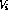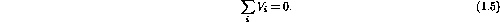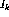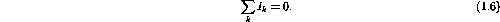Next: Series and Parallel Combinations Up: Direct Current Circuits Previous: Ground

# Kirchoff's Laws

The conservation of energy and conservation of charge when applied to electrical circuits are known as Kirchoff's laws.

Conservation of energy - zero algebraic sum of the voltage dropsaround a closed circuit loop (imaginary loop)Conservation of charge - zero algebraic sum of the currentsflowing into a point (total charge in, equals total charge out)When applying these laws to solve for circuit unknowns we will find the following definitions useful:

• an element is an impedance (resistance) or EMF (ideal voltage source or ideal current source),
• a node is a point where three or more current-carrying elements are connected,
• a branch is one element or several in series connecting two adjacent nodes, and
• an interior loop is a circuit loop not subdivided by a branch.

Using these definitions we can apply Kirchoff's laws to a circuit to solve for the unknown quantities. The general procedure is:

1. define the currents and voltages on a diagram,
2. apply Kirchoff's laws to loops and nodes,
3. write down a set of linear algebraic equations, and
4. solve for the unknowns.

But before we look at general circuits lets consider how simple resistors add.

Doug Gingrich
Tue Jul 13 16:55:15 EDT 1999